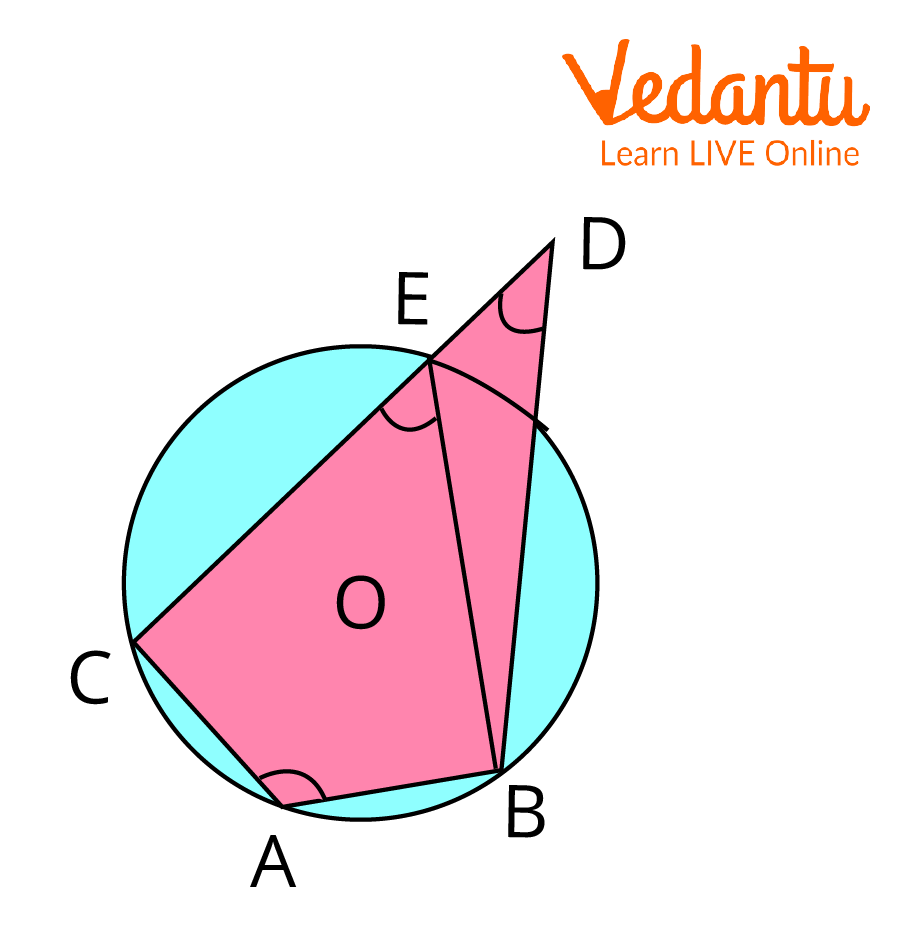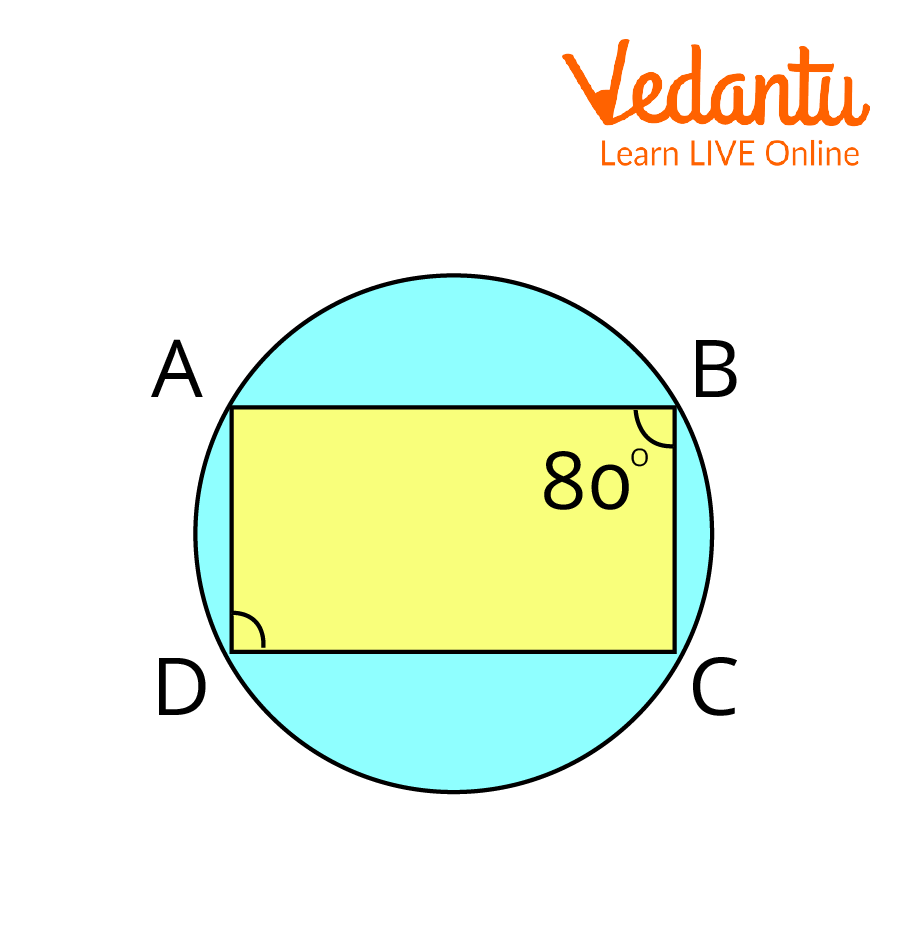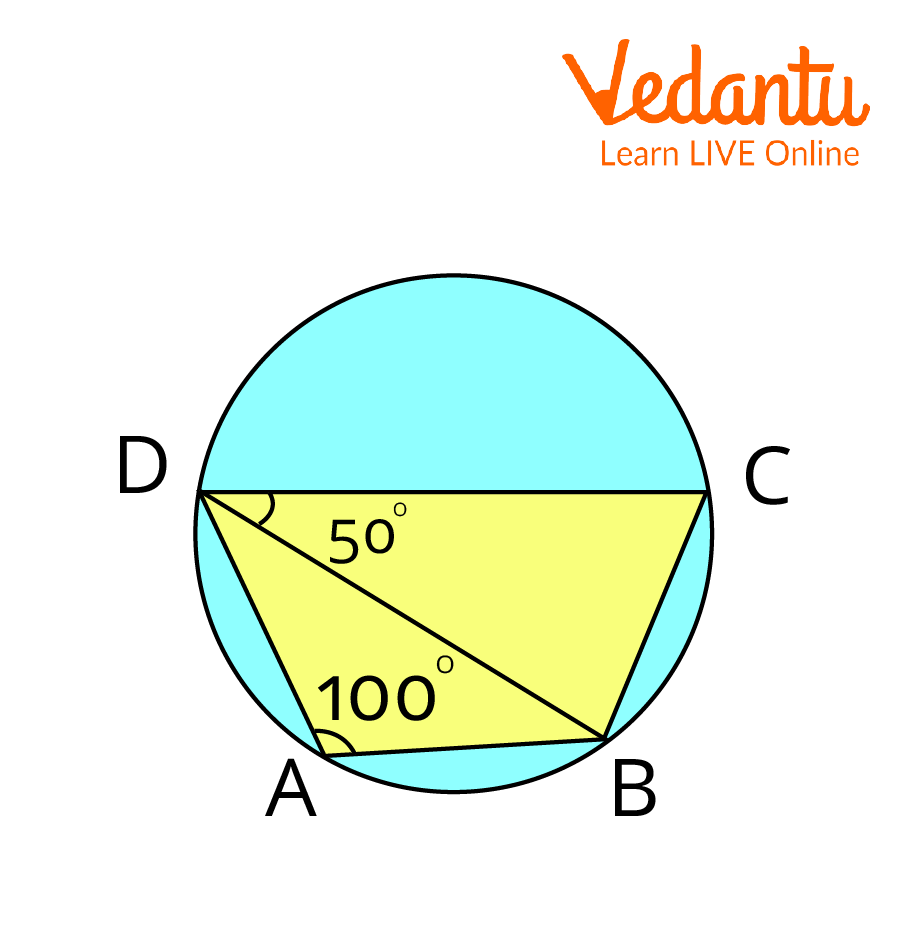Courses
Courses for Kids
Free study material
Free LIVE classes
More

# Theorem on the Sum of Opposite Angles of the Cyclic QuadrilateralLIVE
Join Vedantu’s FREE Mastercalss

## An Overview of the Theorem

In this article, we will prove the theorem and the converse of the theorem on the sum of opposite angles of a cyclic quadrilateral. The Cyclic Quadrilateral Theorem is a fundamental tool of Euclidean Geometry that connects the quadrilateral with the circles and tells us about the properties of cyclic quadrilaterals. In this article, some of the solved examples related to the application of the theorem and the converse of the theorem will also be discussed along with the applications of the theorem in the real world for a crystal clear understanding of the topic.

## History of EuclidEuclid

Name: Euclid

Born:  325 BC

Died:  265 BC

Field: Mathematics

Nationality: Egypt

## Statement of the Theorem on the Sum of Opposite Angles of a Cyclic Quadrilateral

According to the Cyclic Quadrilateral Theorem, the sum of either pair of opposite angles in a cyclic quadrilateral is supplementary, i.e., 180 degrees.

## Proof of Theorem on Sum of Opposite Angles of Cyclic QuadrilateralCyclic quadrilateral ABCD with centre O

Given: Consider a cyclic quadrilateral $A B C D$ in a circle with a centre at $O$.

To prove: $\angle B A D+ \angle B C D=180^{\circ}$

$\angle A B C+ \angle A D C=180^{\circ}$

$A B$ is the chord of the circle.

$\Rightarrow \angle 5=\angle 8$ .. (1) (Angles in the same segment are equal)

$B C$ is the chord of the circle.

$\Rightarrow \angle 1=\angle 6$...(2) (Angles in the same segment are equal)

$C D$ is the chord of the circle.

$\Rightarrow \angle 2=\angle 4 \ldots$... (3) (Angles in the same segment are equal)

$A D$ is the chord of the circle.

$\Rightarrow \angle 7=\angle 3 \quad \ldots$ (4) (Angles in the same segment are equal)

By angle sum property of a quadrilateral,

$\angle A+\angle B+\angle C+\angle D=360^{\circ}$

$\Rightarrow \angle 1+\angle 2+\angle 3+\angle 4+\angle 7+\angle 8+\angle 5+\angle 6=360^{\circ}$

$\Rightarrow(\angle 1+\angle 2+\angle 7+\angle 8)+(3+\angle 4+\angle 5+\angle 6)=360^{\circ}$

$\Rightarrow(\angle 1+\angle 2+7+\angle 8)+(-7+\angle 2+\angle 8+\angle 1)=360^{\circ}$

From equations (1), (2), (3), and (4),

$2(\angle 1+\angle 2+\angle 7+\angle 8)=360^{\circ}$

$\Rightarrow(\angle 1+\angle 2+\angle 7+\angle 8)=180$

$\Rightarrow(\angle 1+\angle 2)+(\angle 7+\angle 8)=180^{\circ}$

$\Rightarrow \angle B A D+\angle B C D=180^{\circ}$

Similarly, $\angle A B C+\angle A D C=180^{\circ}$

Hence proved.

## The Converse of Cyclic QuadrilateralGiven: ABCD is a quadrilateral with:

$\angle B A C+\angle B D C=180^{\circ}$

$\angle A B D+\angle D C A=180^{\circ}$

To Prove: $A B C D$ is a cyclic quadrilateral.

Proof: Since A, B, C are non-collinear, the circle passes through three collinear points.

Let us draw a circle $C_{1}$ with the centre at $O$.

Let us suppose $D$ does not lie on $C_{1}$. Now,

$\therefore A B C E$ is a cyclic quadrilateral.

But given

$\Rightarrow \angle B A C=\angle B E C=180^{\circ}$

Thus,

$\Rightarrow \angle B E C=\angle B D C$

Now, $\ln \Delta \mathrm{BDE}$,

$\Rightarrow \angle B E C=\angle B D E+\angle D B E$ (Exterior angle Property)

$\Rightarrow \angle B E C=\angle B D C+\angle D B E$

$\Rightarrow \angle B D C-\angle B D C=\angle D B E$

$\Rightarrow \angle D B E=0$

$\therefore$ $E$ and $D$ Coincides

Thus, our assumption was wrong.

$\Rightarrow$ Point $D$ lies on circle $C_{1}$

$\Rightarrow A B C D$ is a cyclic quadrilateral.

Hence proved.

## Limitations of the Theorem on the Sum of Opposite Angles of a Cyclic Quadrilateral

• The Cyclic Quadrilateral theorem only tells us about the opposite pair of angles and doesn't tell anything about the corresponding pairs of angles.

• The cyclic quadrilateral is not applicable if any quadrilateral is formed with only three points on the circumference and a fourth point inside the circle.

## Applications of the Theorem on the Sum of Opposite Angles of a Cyclic Quadrilateral

• The cyclic Quadrilateral Theorem is used in computer programming.

• It is used in graphic arts, logos, and packaging.

• It is used in making paintings, sculptures, etc.

## Solved Examples

1. Find the value of angle D of a cyclic quadrilateral, if angle B is $80^{\circ}$.ABCD is a cyclic quadrilateral with angle B is 80 degrees.

Ans: Since $A B C D$ is a cyclic quadrilateral,

Hence, the sum of a pair of two opposite angles $=180^{\circ}$.

$\Rightarrow \angle B+\angle D=180$

$\Rightarrow 80^{\circ}+\angle D=180^{\circ}$

$\Rightarrow \angle D=180^{\circ}-80^{\circ}$

$\Rightarrow \angle D=100^{\circ}$

The value of angle $D$ is $100^{\circ}$.

2. Find the value of angle $D$ of a cyclic quadrilateral, if angle $B$ is $120^{\circ}$.
Ans:
As ABCD is a cyclic quadrilateral, hence the sum of a pair of two opposite angles $=180^{\circ}$.

$\Rightarrow \angle B+\angle D=180^{\circ} \\$

$\Rightarrow 120^{\circ}+\angle D=180^{\circ} \\$

$\Rightarrow \angle D=180^{\circ}-120^{\circ} \\$

$\Rightarrow \angle D=60^{\circ}$

The value of angle D is $60^{\circ}$.

3. In the figure given below, $A B C D$ is a cyclic quadrilateral in which

$\angle B A D=100^{\circ}$ and $\angle C D B=50^{\circ}$. find $\angle D B C$ ?To find angle DBC in a cyclic quadrilateral

Ans:

Given,

$\angle B A D=100^{\circ}$ and $\angle C D B=50^{\circ}$

$\angle B A D+\angle B C D=180^{\circ}$ as these both angles are opposite angles of a cyclic quadrilateral.

$\Rightarrow \angle B C D=180^{\circ}-100^{\circ}=80^{\circ}$

In $\triangle B C D$,
$\angle B C D+\angle C D B+\angle D B C=180^{\circ}$

$\Rightarrow 80^{\circ}+50^{\circ}+\angle D B C=180^{\circ}$

$\Rightarrow \angle D B C=180^{\circ}-130^{\circ}$

$\Rightarrow \angle D B C=50^{\circ}$

Therefore,

$\Rightarrow \angle D B C=50^{\circ}$

## Important Points to Remember

• The sum of pairs of opposite angles of a cyclic quadrilateral is always supplementary.

• If the sum of a pair of opposite angles of a Quadrilateral is supplementary, then the quadrilateral is a cyclic quadrilateral.

## Important Formulas to Remember

• If $ABCD$ is a cyclic quadrilateral in a circle with centre O, then $\angle B A D+ \angle B C D=180^{\circ}$.

• If in a quadrilateral $ABCD$, $\angle B A D+ \angle B C D=180^{\circ}$, then $ABCD$ must be a cyclic quadrilateral.

## Conclusion

In the article, we have discussed the proof of the Cyclic Quadrilateral Theorem and its converse. Applications of Cyclic Quadrilaterals are also discussed in this article. Cyclic Quadrilaterals are connecting links between polygons and circles. In all, we can say that the Cyclic Quadrilaterals are very important component of Geometry and connects the fundamental tools of geometry, i.e., Polygons and circles.

Last updated date: 03rd Oct 2023
Total views: 104.1k
Views today: 3.04k

## FAQs on Theorem on the Sum of Opposite Angles of the Cyclic Quadrilateral

1. What is meant by Cyclic Quadrilaterals?

The quadrilaterals are the figures which have 4 sides and any quadrilateral is said to be cyclic quadrilateral if all the vertices of the quadrilateral lie on the circumference of the circle. Cyclic Quadrilaterals are the special type of quadrilaterals in Euclidean Geometry which connects the quadrilateral figures with the circle. If there is a quadrilateral whose one or more sides are inside the circle and not on the circumference of the circle, such type of quadrilateral cannot be called a cyclic quadrilateral.

2. What are the properties of Cyclic Quadrilaterals?

Some of the properties of Cyclic Quadrilaterals are as follows:

• The vertices of the cyclic quadrilateral always lie on the circumference of the circle.

• The area of the Cyclic Quadrilaterals is calculated using Heron's formula.

• The Perpendicular bisectors in Cyclic Quadrilateral are always concurrent.

• The Perpendicular bisectors of the sides of the Cyclic Quadrilateral meet at the centre of the circle.

• If any side of the cyclic quadrilateral is extended, then the exterior angle formed is equal to the sum of interior opposite angles.

3. What are concyclic points and how are they related to cyclic quadrilaterals?

The points are said to be concyclic points if they lie on the same circle or we can say that if a circle passes through all the points, then the points are said to be concyclic. An interesting property regarding concyclic points is that any three noncollinear points are always concyclic as there will always exist a circle passing through such points. The polygon formed using concyclic points is called a cyclic polygon. If there are four concyclic points, then the polygon formed using four concyclic points is called a cyclic quadrilateral.